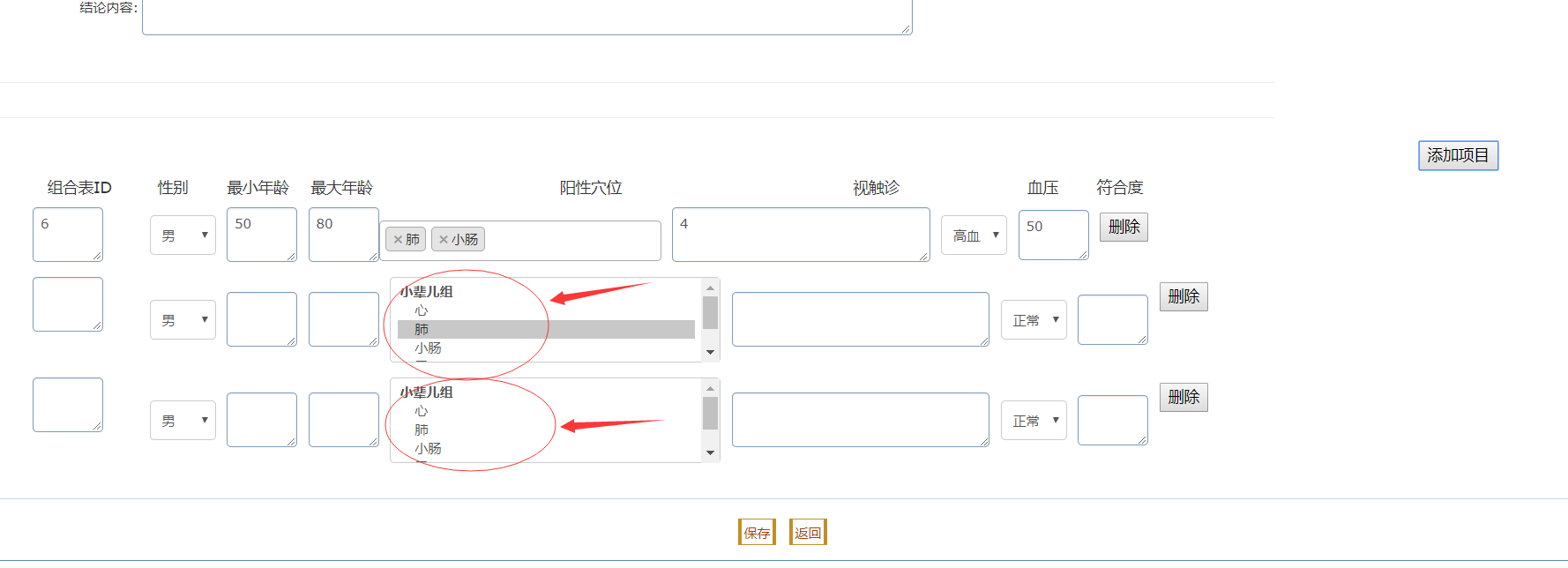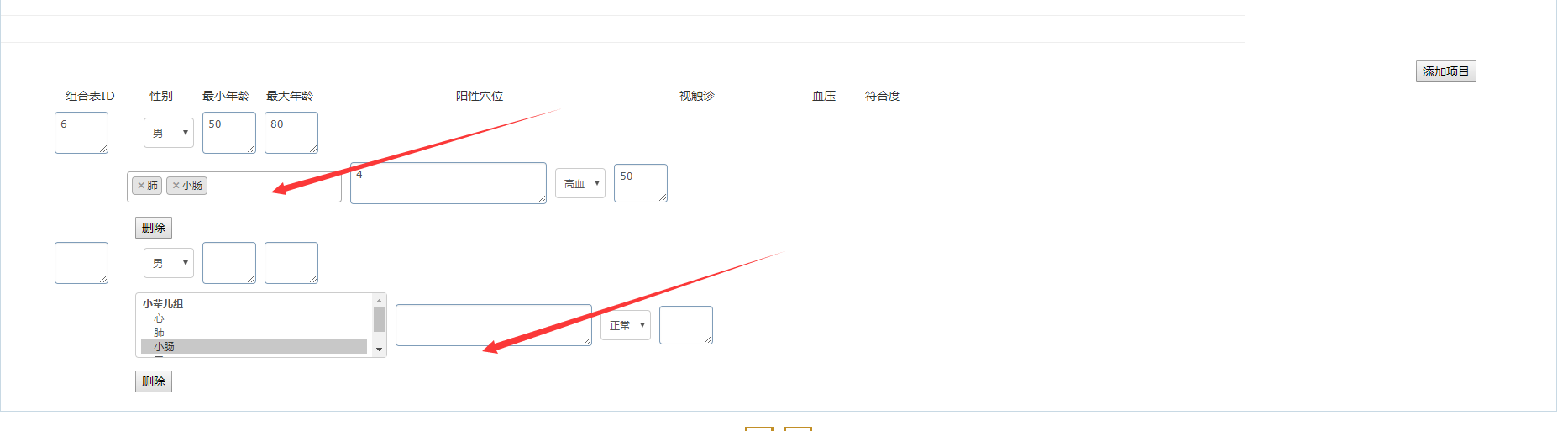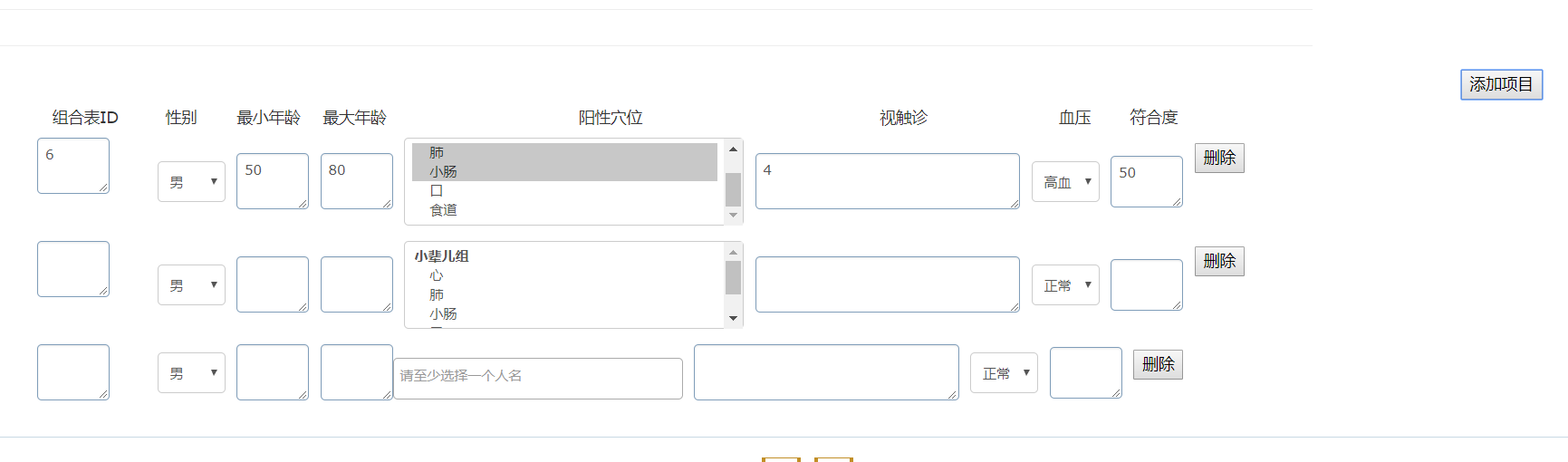###### bulidfer

2018-07-31 18:01 阅读 2.6k

# select2 用js动态循环添加 没有任何渲染 只有第一个默认有渲染

20js代码

`````` function updateView(id, system_id) {
\$("#btntype").val(1);

\$("#Submit1").click(function() {
add(1);

});

\$
.ajax({
type : "POST",
url : "/erzhentang/manage/forAjax.do?requestType=12.1",
data : "id=" + id + "&system_id=" + system_id, // 发送到服务器的数据默认id是结论id
success : function(msg) {
\$("#xuewei_id").val("1,2,3").select2();
/* alert(msg); */
var json = eval('(' + msg + ')');
document.getElementById("addModel").style.display = "block";
document.getElementById("id1").value = json.earScanJielun.id;
document.getElementById("system_id1").value = json.earScanJielun.system_id;
/* 遍历所属系统下所有 耳穴频扫疾病项 */
var arrESJ = eval(json.listEarScanJibing);// arrESJ是一个数组
var checkESJ = document.getElementById("jibing_num1");
var str2 = "";
for (var j = 0; j < arrESJ.length; j++) {
str2 += "<option value=\"" + arrESJ[j].jibing_num
+ "\">" + arrESJ[j].name + "</option>";
var checkESJ2 = arrESJ[j].name;
if (arrESJ[j].jibing_num == json.earScanJielun.jibing_num) {// 疾病编号人为控制每个编号都是唯一
arrESJ[j].name = "selected='selected'";
}
\$("#jibing_num1").html(str2);
}
document.getElementById("jibing_num1").value = json.earScanJielun.jibing_num;
document.getElementById("jielun_rank1").value = json.earScanJielun.jielun_rank;
document.getElementById("jielun1").value = json.earScanJielun.jielun;
/* 下半截部分组合表动态展示修改和添加 */
var arrESC = eval(json.listEarScanCp);// arrESC是一个数组频扫结论组合表
var str = "";
var str1 = "";
for (var i = 0; i < arrESC.length; i++) {
str += "<tr>";
str += "<td colspan=\"8\">";
str += "<div style=\"margin-left: 148px;\" id=\"earScanCp\">";
str += "<div style=\"float: left;\">";
str += "<textarea style=\"width: 50px;margin-left: -86px;position: absolute;\" id=\"cp_id\" name=\"cp_id\" >"
+ arrESC[i].id + "</textarea>";
str += "<select style=\"width: 60px;height: 36px;margin-left: 20px;\" id=\"gender\" name=\"gender\" >"
var myselect = document.getElementById("gender");// 这个获取的是静态jsp页面里标签

str += "<select style=\"width: 300px;margin-left:10px;\" id=\"xuewei_id\" name=\"xuewei_id\"class=\"combox\" multiple >"
str += "<optgroup label=\"小辈儿组\">";
str += "<option value=\"1\">心</option>";
str += "<option value=\"2\" " + "selected='selected'"
+ ">" + "肺" + "</option>";
str += "<option value=\"3\" " + "selected='selected'"
+ ">" + "小肠" + "</option>";
str += "<option value=\"4\">" + "口" + "</option>";
str += "<option value=\"5\">" + "食道" + "</option>";
str += "</optgroup>";

"</select>";

str += "</div>";
str += "<div >";
str += " <input type=\"button\" onclick=\"delRow(this,"
+ arrESC[i].id
+ ","
+ arrESC[i].bianzhengId
+ ")\" value=\"删除\" id=\"deleteESC\" name=\"deleteESC\" style=\"float: left; margin-left:10px; margin-top: 5px;\">";
str += "</div>";
str += "</div>";
str += "</td>";
str += "</tr>";
/* 替换静态页面 */
\$("#tbody1").html(str);

select2Update();//执行select方法
}

}
});
}
``````

``````

function addRow1() {
var str = "";
str += "<tr>";
str += "<td colspan=\"8\">";
str += "<div style=\"margin-left: 148px;\" id=\"earScanCp\">";
str += "<div style=\"float: left;\">";
str += "<textarea style=\"width: 50px;margin-left: -86px;position: absolute;\" id=\"cp_id\" name=\"cp_id\" ></textarea>";
str += "<select style=\"width: 60px;height: 36px;margin-left: 20px;\" id=\"gender\" name=\"gender\" >"
var myselect = document.getElementById("gender");// 这个获取的是静态jsp页面里标签
for (var j = 0; j < myselect.options.length; j++) {// 下拉框是主家从静态页面获取并且遍历它
str += "<option value=\"" + myselect.options[j].value + "\">"
+ myselect.options[j].text + "</option>";
}
"</select>";
str += "<textarea style=\"width: 50px;margin-left: 10px;\" id=\"min_age\" name=\"min_age\" ></textarea>";
str += "<textarea style=\"width: 50px;margin-left: 10px;\" id=\"max_age\" name=\"max_age\" ></textarea>";
// str += "<textarea style=\"width: 300px;margin-left: 10px;\"
// id=\"xuewei_id\" name=\"xuewei_id\" ></textarea>";
str += "<select style=\"width: 300px;margin-left:10px;\" id=\"xuewei_id\" name=\"xuewei_id\" class=\"combox\" multiple >"
str += "<optgroup label=\"小辈儿组\">";
str += "<option value=\"1\">心</option>";
str += "<option value=\"2\">" + "肺" + "</option>";
str += "<option value=\"3\">" + "小肠" + "</option>";
str += "<option value=\"4\">" + "口" + "</option>";
str += "<option value=\"5\">" + "食道" + "</option>";
str += "</optgroup>";
"</select>";

str += "<textarea style=\"width: 220px;margin-left: 10px;\" id=\"chuzhen_id\" name=\"chuzhen_id\" ></textarea>";
str += "<select style=\"width: 60px;height: 36px;margin-left: 10px;\" id=\"bp\" name=\"bp\" >"
var myselect1 = document.getElementById("bp");// 这个获取的是静态jsp页面里标签
for (var k = 0; k < myselect1.options.length; k++) {// 下拉框是主家从静态页面获取并且遍历它
// alert("j值：" + myselect1.options[k].value);
// alert("text值：" + myselect1.options[k].text);
str += "<option value=\"" + myselect1.options[k].value + "\">"
+ myselect1.options[k].text + "</option>";
}
"</select>";
str += "<textarea style=\"width: 50px; height: 36px;margin-left: 10px;\" id=\"jielun_conform\" name=\"jielun_conform\"></textarea>";
str += "</div>";
str += "<div >";
str += " <input type=\"button\" onclick=\"delRow(this)\" value=\"删除\" id=\"deleteESC\" name=\"deleteESC\" style=\"float: left; margin-left:10px; margin-top: 5px;\">";
str += "</div>";
str += "</div>";
str += "</td>";
str += "</tr>";
\$("#rowTable1").append(str);
// \$("select[name='xuewei_id']").select2({
//  \$("#xuewei_id").select2().trigger();
\$("#xuewei_id").trigger('change')
select2Save();
}

``````

select2方法 包含添加和修改 目前两个方法完全一致

`````` function select2Update() {
\$("#xuewei_id").trigger('change')
\$(function() {
\$('#xuewei_id').select2({
placeholder : "请至少选择一个人名",
tags : true,
createTag : function(decorated, params) {
return null;
},
width : '256px'
});

function formatState(state) {
if (!state.id) {
return state.text;
}
var \$state = \$('<span>' + state.text + '</span>');
return \$state;
}
;

\$('#sel_recommender').select2({
placeholder : "请选择一个人名",
templateResult : formatState,
width : '256px'
});
});
}

function select2Save() {

\$(function() {
//       \$("select[name='xuewei_id']").select2({
\$('#xuewei_id').select2({
placeholder : "请至少选择一个人名",
tags : true,
createTag : function(decorated, params) {
return null;
},
width : '256px'
});

function formatState(state) {
if (!state.id) {
return state.text;
}
var \$state = \$('<span>' + state.text + '</span>');
return \$state;
}
;

\$('#sel_recommender').select2({
placeholder : "请选择一个人名",
templateResult : formatState,
width : '256px'
});
});
``````
• 点赞
• 写回答
• 关注问题
• 收藏
• 复制链接分享

#### 3条回答默认 最新

• 已采纳

id是唯一的，不建议一个页面有多个相同的id。虽然不会报错但有反规范，楼上兄弟做法可以解决，重复id最好还是不用id选择器，建议你用类选择器，除了老板的ie浏览，现在的新浏览器用类选择器性能低于id选择器而高于其它
选择器，比如属性选择器。给你个例子：

``````<div id="selectSection">
<select class="select2" name="xx1"/>
<select class="select2" name="xx1"/>
</div>

\$("#selectSection").find("select.select2").select2();
或\$("#selectSection select.select2").select2();
或\$("select.select2， #selectSection").select2();
或\$("select.select2").select2();
``````
点赞 评论 复制链接分享
• id不能重复，要不id选择器只会选出第一个dom，所以就是你这个问题，只有第一个dom被选出来进行初始化select2，改为属性选择器

`````` \$('select[id="xuewei_id"]').select2();
``````
点赞 评论 复制链接分享
•点赞 评论 复制链接分享